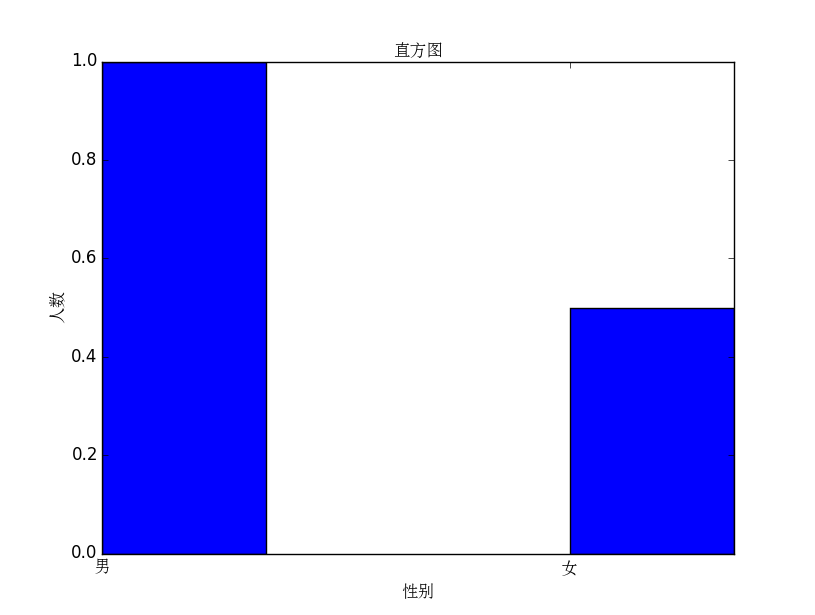# Python 3下Matplotlib画图中文显示乱码的解决方法

Matplotlib是Python的一个很好的绘图包，但是其本身并不支持中文（貌似其默认配置中没有中文字体），所以如果绘图中出现了中文，就会出现乱码。

# 最小二乘拟合示例
import numpy as np
from scipy.optimize import leastsq
import matplotlib.pyplot as plt
import matplotlib

def func(x, p):
"""
数据拟合所用的函数: A*sin(2*pi*k*x + theta)
"""
A, k, theta = p
return A*np.sin(2*np.pi*k*x+theta)

def residuals(p, y, x):
"""
实验数据x, y和拟合函数之间的差，p为拟合需要找到的系数
"""
return y - func(x, p)

x = np.linspace(0, -2*np.pi, 100)
A, k, theta = 10, 0.34, np.pi/6 # 真实数据的函数参数
y0 = func(x, [A, k, theta]) # 真实数据
y1 = y0 + 2 * np.random.randn(len(x)) # 加入噪声之后的实验数据

p0 = [7, 0.2, 0] # 第一次猜测的函数拟合参数

# 调用leastsq进行数据拟合
# residuals为计算误差的函数
# p0为拟合参数的初始值
# args为需要拟合的实验数据
plsq = leastsq(residuals, p0, args=(y1, x))

print("真实参数:", [A, k, theta])
print("拟合参数", plsq) # 实验数据拟合后的参数

plt.plot(x, y0, label="真实数据")
plt.plot(x, y1, label="带噪声的实验数据")
plt.plot(x, func(x, plsq), label="拟合数据")
plt.legend()
plt.savefig('fit.jpg')
plt.show()

真实参数: [10, 0.34, 0.5235987755982988]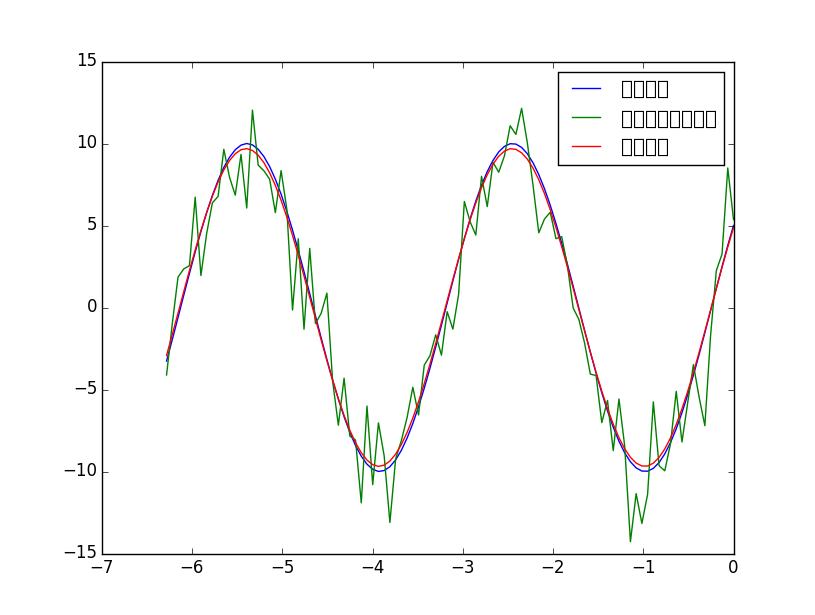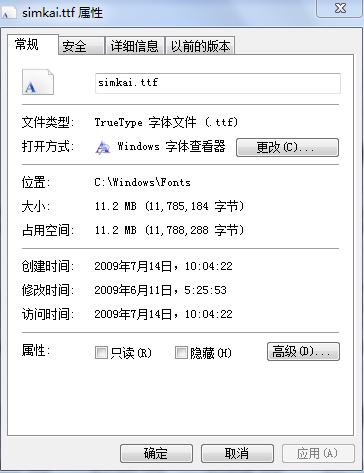zhfont1 = matplotlib.font_manager.FontProperties(fname='C:\Windows\Fonts\simkai.ttf')

plt.legend(prop=zhfont1)

# 最小二乘拟合示例
import numpy as np
from scipy.optimize import leastsq
import matplotlib.pyplot as plt
import matplotlib

zhfont1 = matplotlib.font_manager.FontProperties(fname='C:\Windows\Fonts\simkai.ttf')

def func(x, p):
"""
数据拟合所用的函数: A*sin(2*pi*k*x + theta)
"""
A, k, theta = p
return A*np.sin(2*np.pi*k*x+theta)

def residuals(p, y, x):
"""
实验数据x, y和拟合函数之间的差，p为拟合需要找到的系数
"""
return y - func(x, p)

x = np.linspace(0, -2*np.pi, 100)
A, k, theta = 10, 0.34, np.pi/6 # 真实数据的函数参数
y0 = func(x, [A, k, theta]) # 真实数据
y1 = y0 + 2 * np.random.randn(len(x)) # 加入噪声之后的实验数据

p0 = [7, 0.2, 0] # 第一次猜测的函数拟合参数

# 调用leastsq进行数据拟合
# residuals为计算误差的函数
# p0为拟合参数的初始值
# args为需要拟合的实验数据
plsq = leastsq(residuals, p0, args=(y1, x))

print("真实参数:", [A, k, theta])
print("拟合参数", plsq) # 实验数据拟合后的参数

plt.plot(x, y0, label="真实数据")
plt.plot(x, y1, label="带噪声的实验数据")
plt.plot(x, func(x, plsq), label="拟合数据")
plt.legend(prop=zhfont1)
plt.savefig('fit.jpg')
plt.show()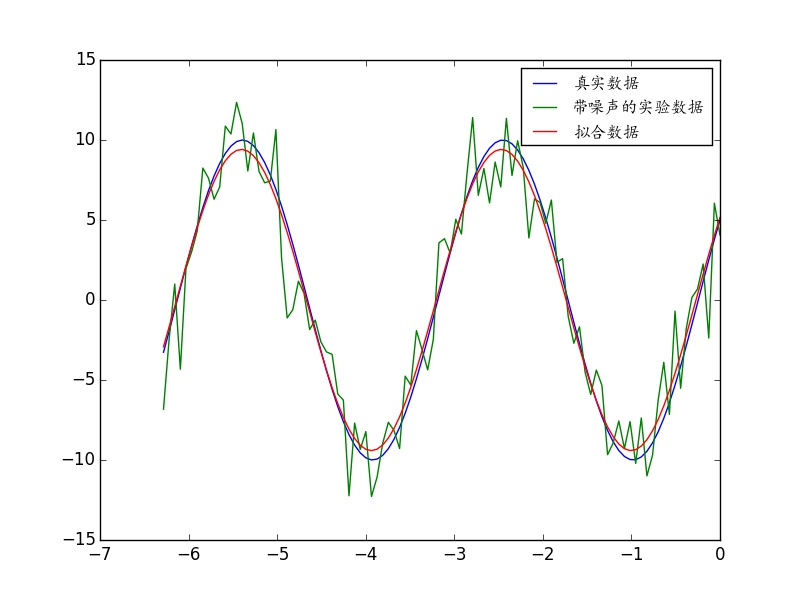plt.savefig('fit.pdf')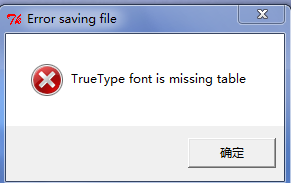E:\Program Files\Python\Lib\site-packages\matplotlib\mpl-data\fonts\ttf

#中文标题与坐标轴示例
import matplotlib.pyplot as plt
import matplotlib
zhfont1 = matplotlib.font_manager.FontProperties(fname='C:\Windows\Fonts\simsun.ttc')
plt.xlabel('性别',fontproperties=zhfont1)
plt.ylabel('人数',fontproperties=zhfont1)
plt.title('直方图',fontproperties=zhfont1)
plt.xticks( (0,1),('男','女') ,fontproperties=zhfont1)
plt.bar(left=(0,1), height=(1,0.5), width=0.35)
plt.show()